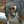# Correctly truncating a binary counter sequenceKen Yap wrote 07/18/2019 at 02:23 • 2 min read • Like

I was working with a 7493 counter last night. This is a TTL 4-bit counter with a two independent sections, a divide by 2 and a divide by 8. It also has two reset to 0 pins which are ANDed. You can use it as a divide by N counter where N is less than 16, provided N doesn't have more than two 1 bits in the binary representation. So to divide by 6 you would reset at 110.

I wanted to divide by 12 so I decided to divide by 6, then divide by 2, so that I would have a 50% duty cycle waveform on the last output. So I connected the reset pins to bits 1 and 2.

The first section did divide by 6, but the second section stayed at 0. I tried several chips with the same symptom so not just one bad chip. What was happening?

After a while I realised that the count sequence was:

0000, 0001, 0010, 0011, 0100, 0101, 0110 -> 0000

So the divide by 2 section never reached 1.

The correct way is to divide by 2, then divide by 6, and to connect the reset to bits 2 and 3. The sequence then becomes:

0000, 0001, 0010, 0011, 0100, 0101, 0110, 0111, 1000, 1001, 1010, 1011, 1100 -> 0000

I have to give up the 50% duty cycle, but that's cosmetic. My mistake was taking the meaning of independent too far. The counters do work independently except for the reset function. Sometimes concepts can be oversimplified.

## Discussions